# GATE 2021 AR

This page will get populated over time

How was GATE 2021 AR? Short Polls:

Was GATE AR 2021 difficult than the GATE AR 2020?

• Yes (69%, 318 Votes)
• No (31%, 144 Votes)

Total Voters: 462Loading ...

What do you think the expected cut-off would be?

• 33 to 35 (61%, 300 Votes)
• 36 to 40 (22%, 109 Votes)
• 41 to 45 (17%, 83 Votes)

Total Voters: 492Loading ...

The cut-off is surged to 40.9. What could be the reason?

View ResultsLoading ...

#### Question & Answer GATE 2021

Aptitude Section

Q1.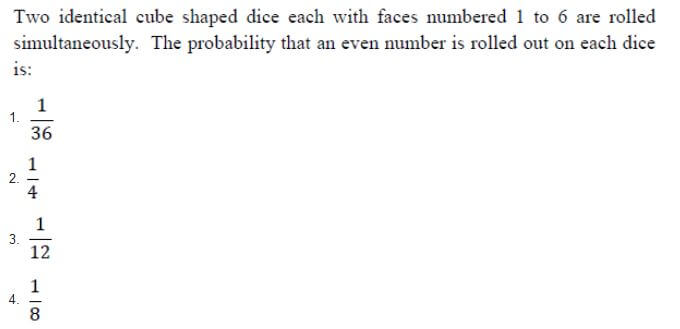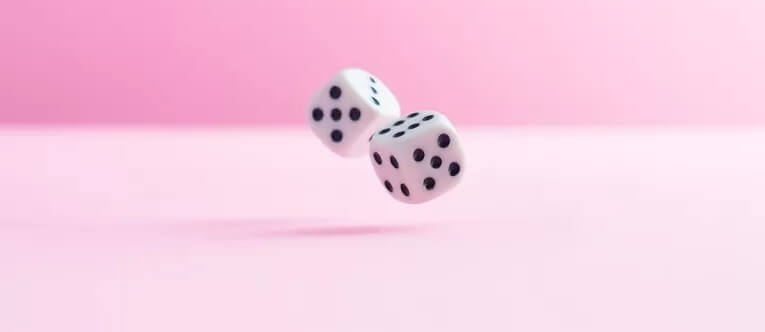Solution: Required probability = P(both are even) = P(first is even) * P(second is even) = (3/6)*(3/6) = (1/2)*(1/2)
= 1/4 Answer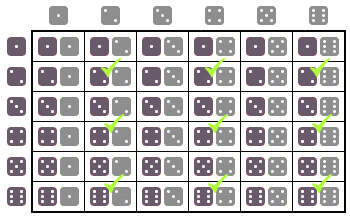Q2.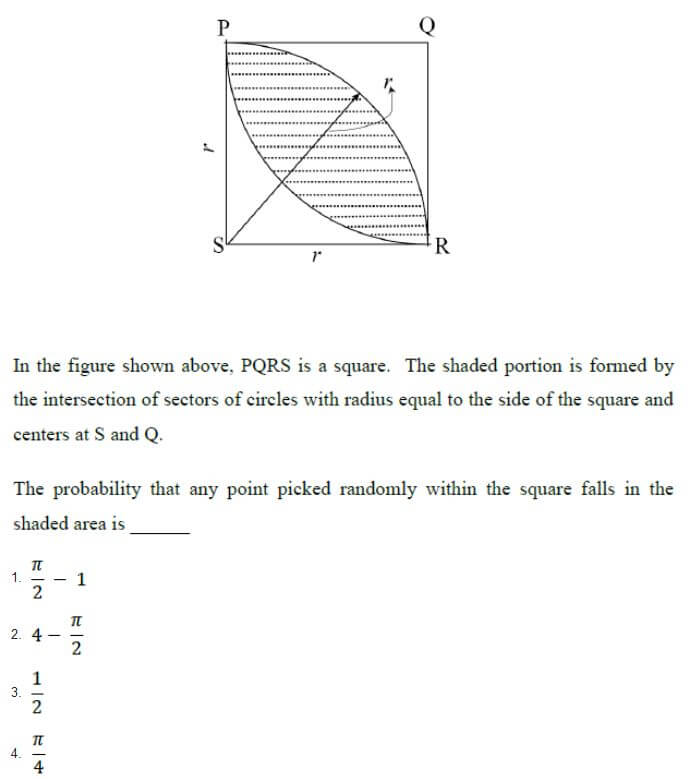Solution: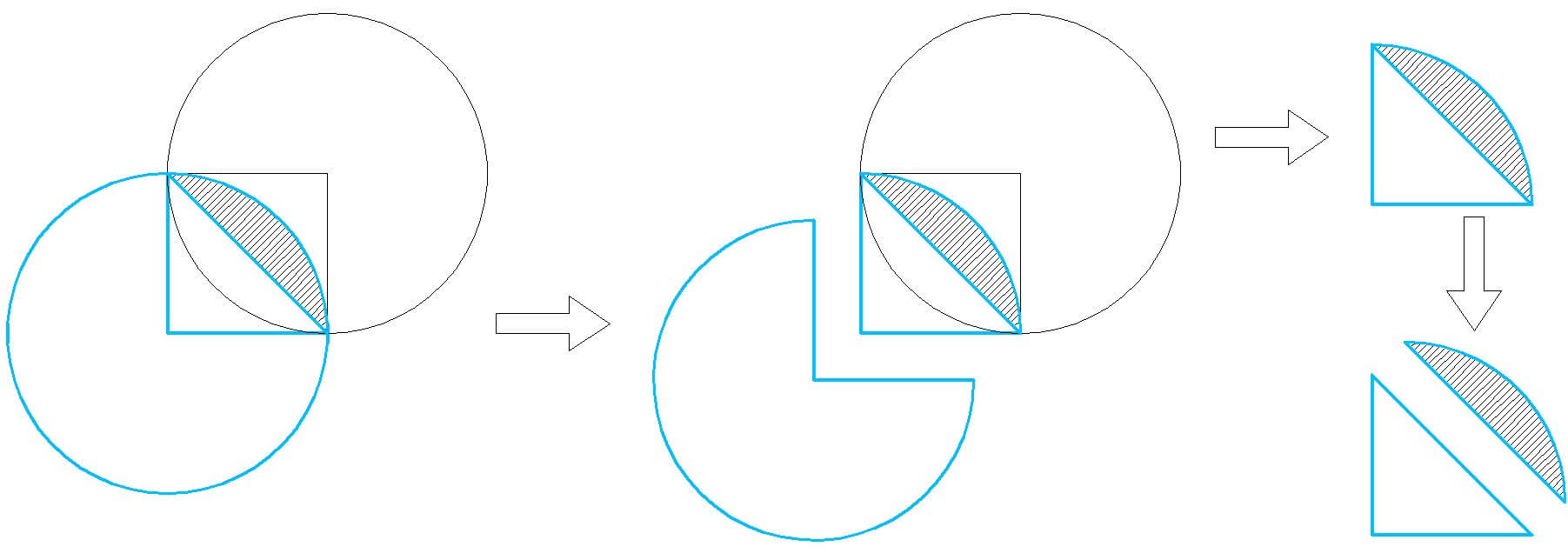The hatched region is called Secant of a circle. Area of secant = 1/4(Area of circle) – Area of triangle shown
= 1/4 (π r2) – 1/2 (base*height) = 1/4 (π r2) – 1/2 (r*r) = 1/4 (π r2) – 1/2 (r2) = 1/2(π/2 – 1)         [r = 1 given]There are two such Secants, So, area of dotted region surrounded by Secants shown in the question = 2*1/2(π/2 – 1)
= π/2 – 1
Area of square = r*r = 1*1 = 1

Therefore, Required probability = Area surrounded by Secants / Area of square = (π/2 – 1) / 1 = π/2 – 1 Answer

Q3.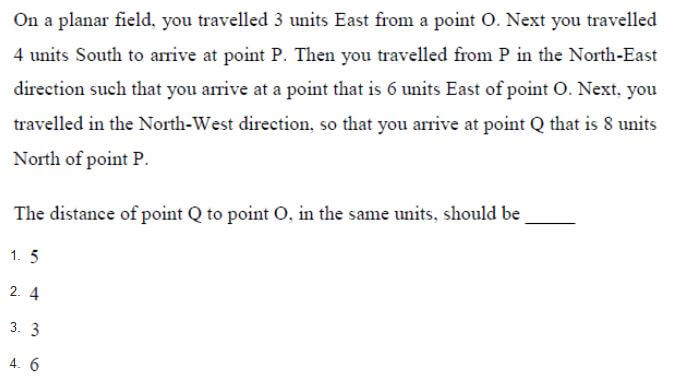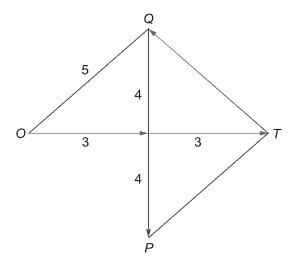Answer: (1)

Q4.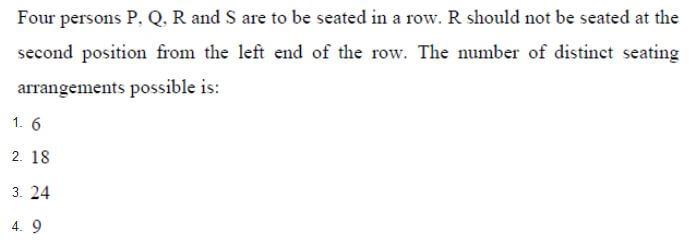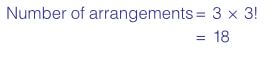Q5.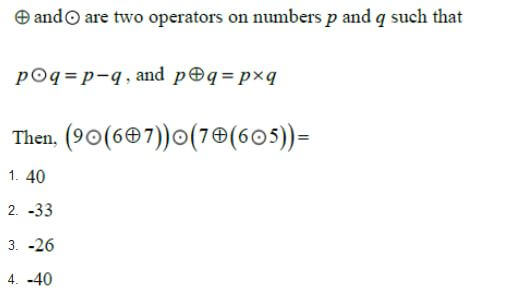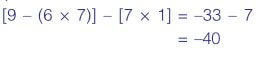Q6.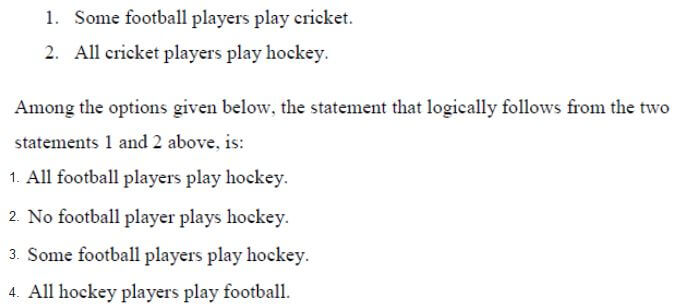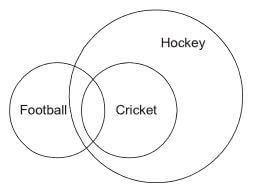Answer: (3)

Q7.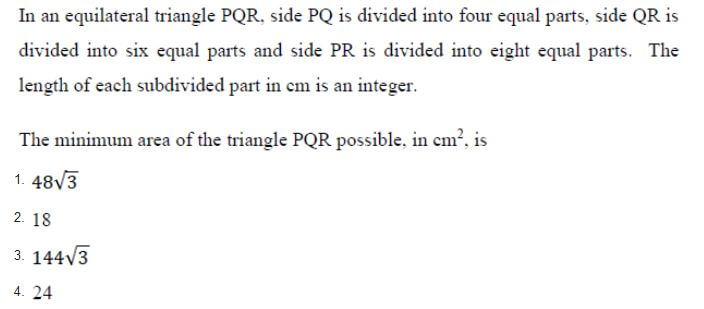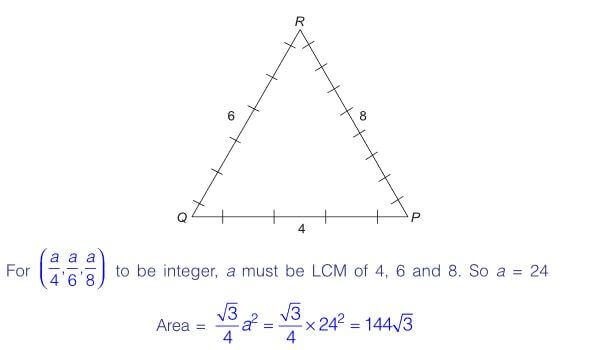Q8.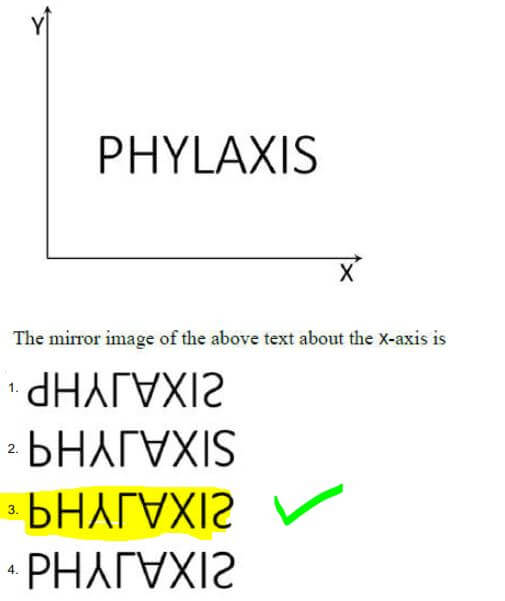Q9.Q10.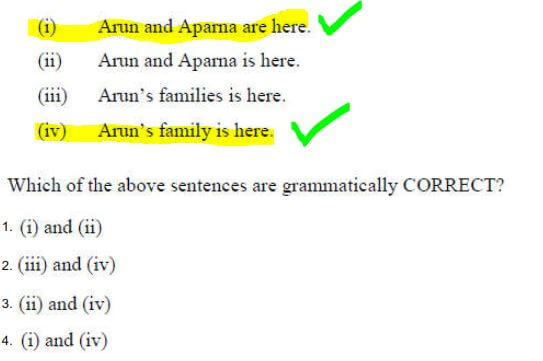Answer: (4)

Architecture & Planning Section

Q11.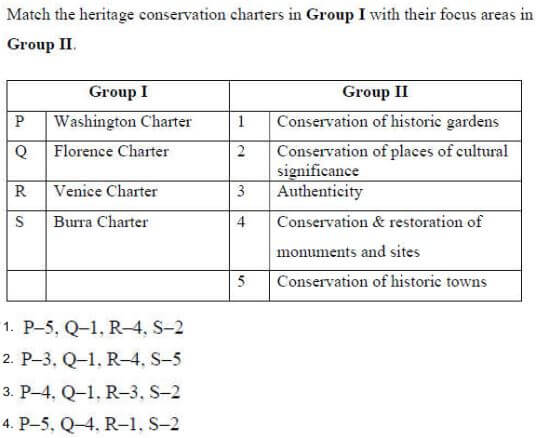Answer: (1)

Q12.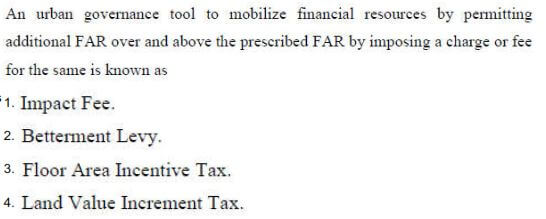Answer: (3)

Q13.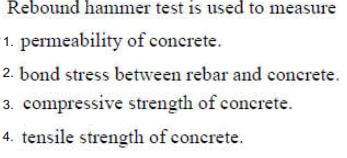Answer: (3)

Q14.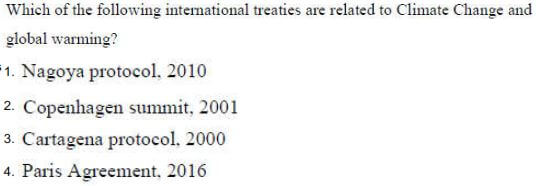Answer: (4)

Q15.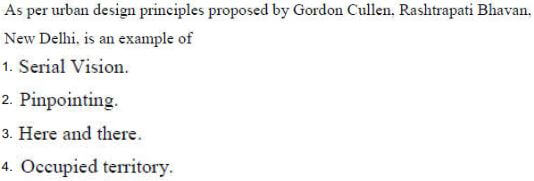Answer: (1)

Q16.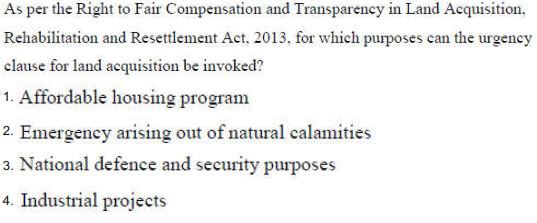Answer: (3) Invoke = Apply or implement, Revoke = cancel

Q17.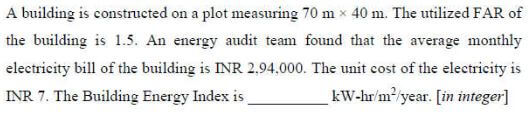Q18.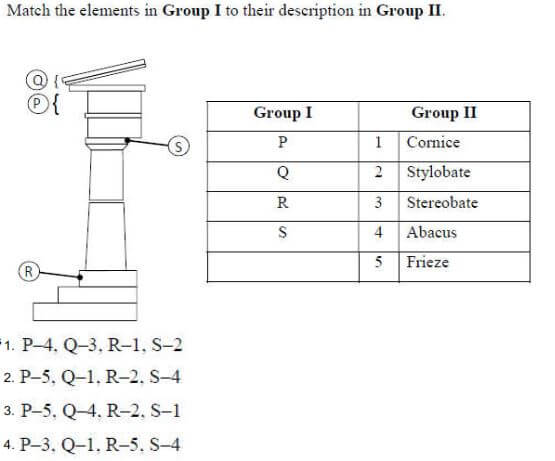Answer: (2)

Q19.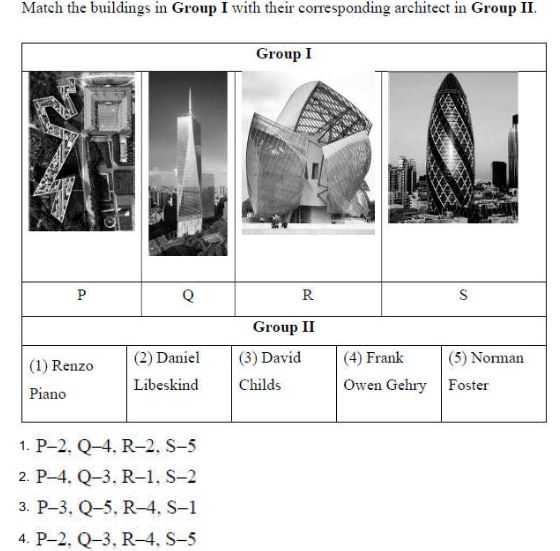Answer: (4)

Q20.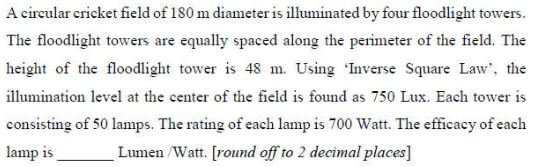Q21. (MSQ Type)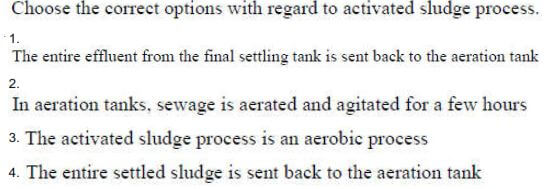Q22.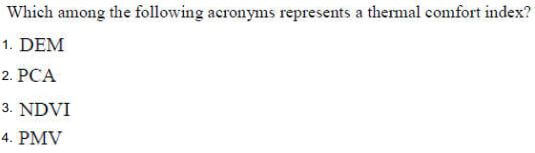Q23.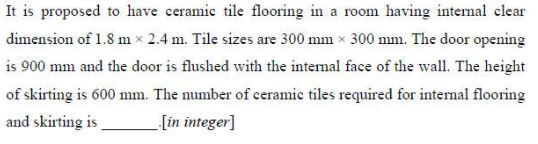Q24.Q25.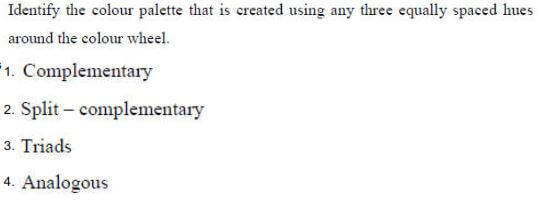Q26.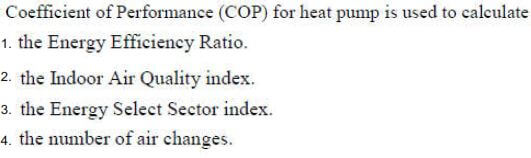Q27.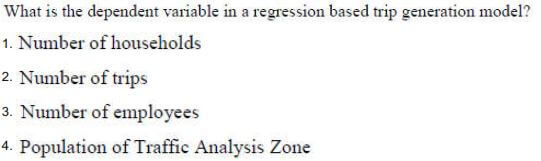Q28.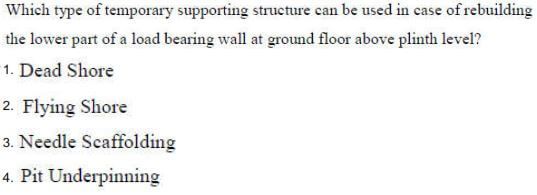Q29.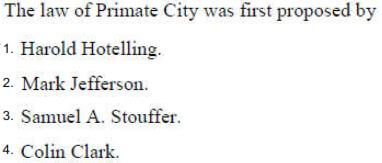Answer: (2)

Q30. (MSQ Type)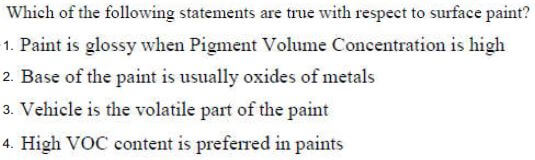Q31.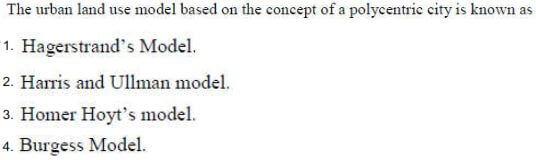Q32.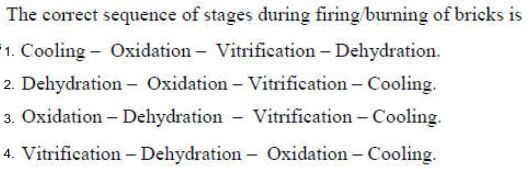Q33.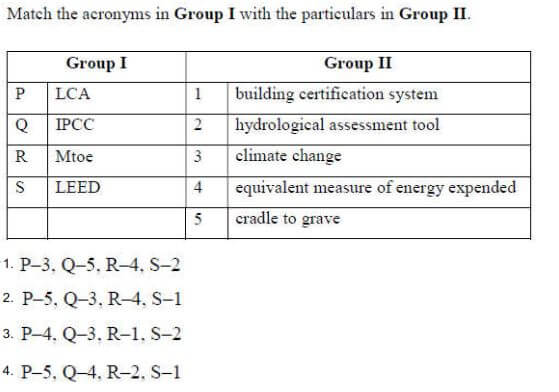Q34.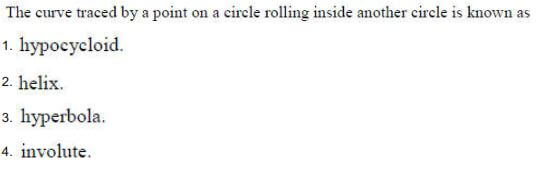Q35.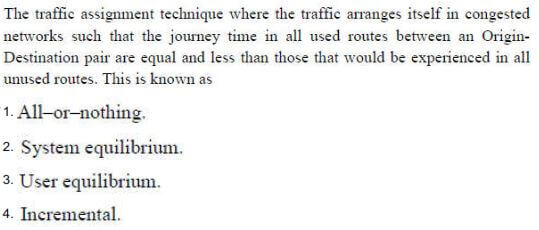Q36.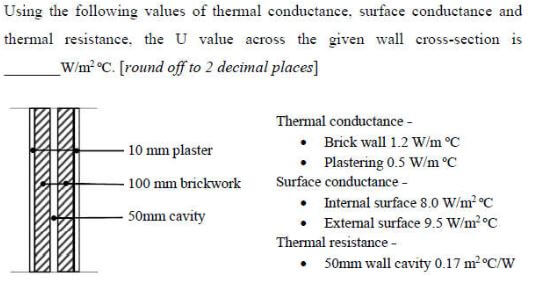Q37.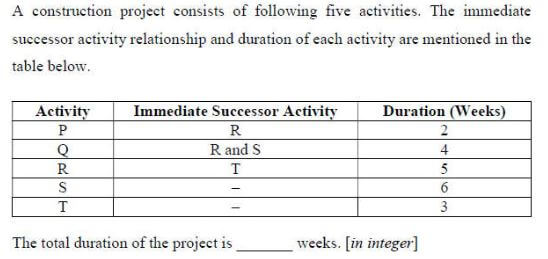Q38.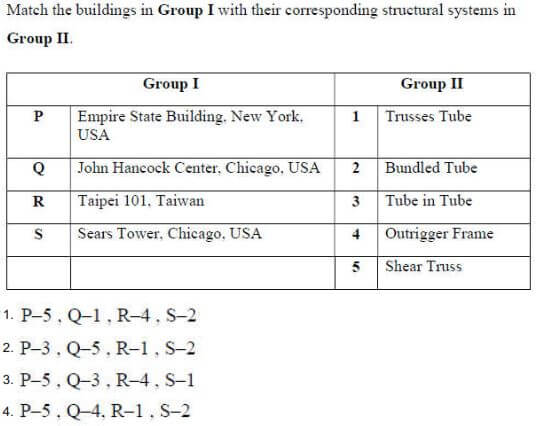Answer: (1)

Q39.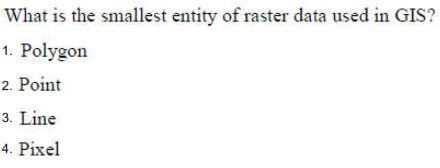Answer: (4)

Q40.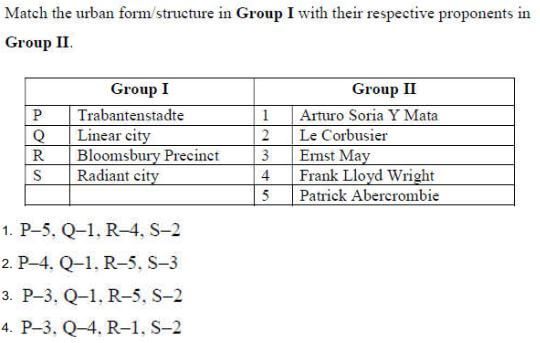Q41.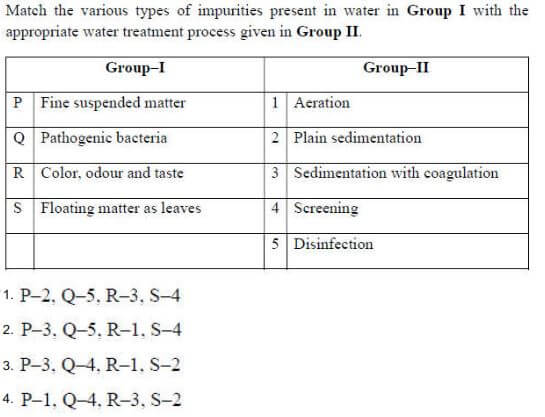Q42.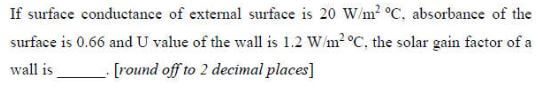Q43.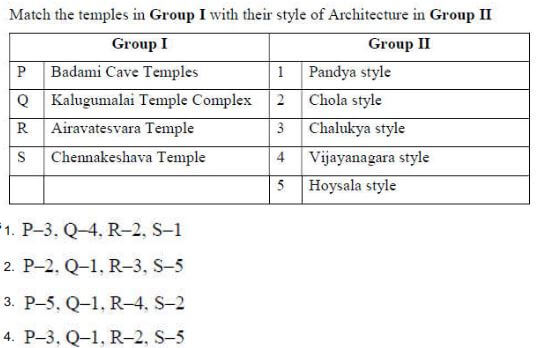Q44.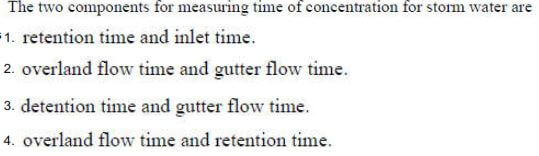Q45.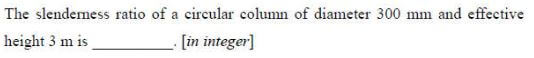Q46.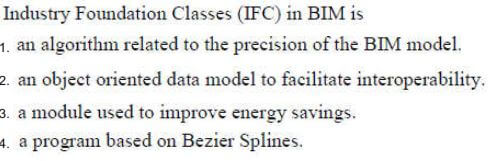Q47.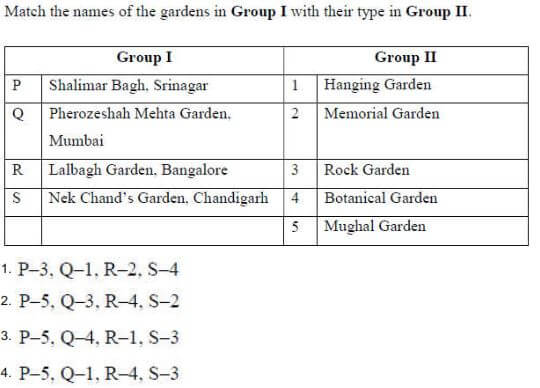Q48.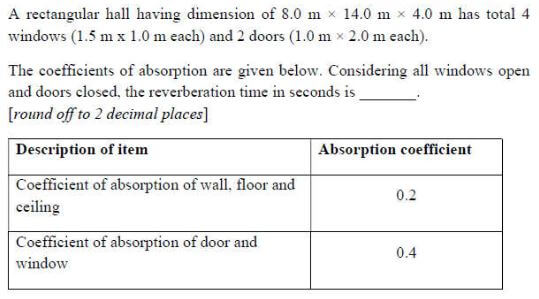Q49. (MSQ Type)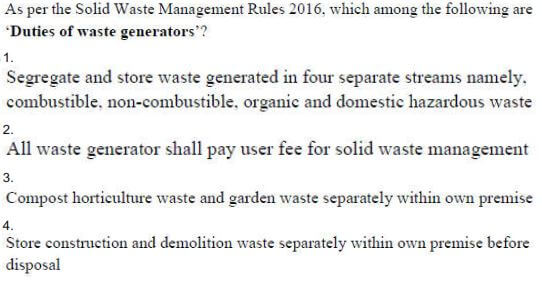Q50.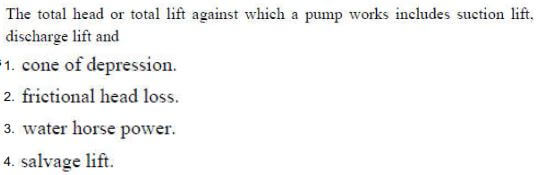Q51.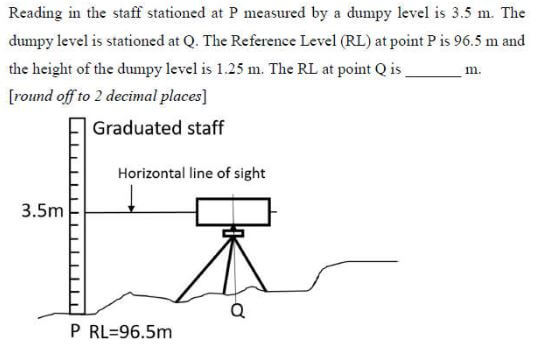Q52.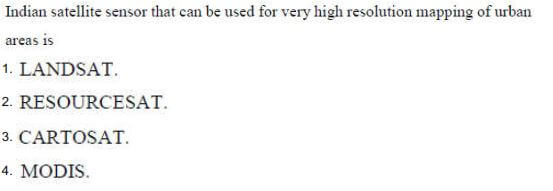Q53.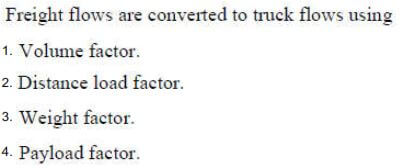Q54.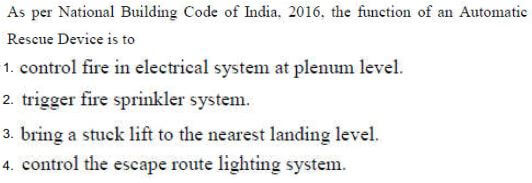Q55.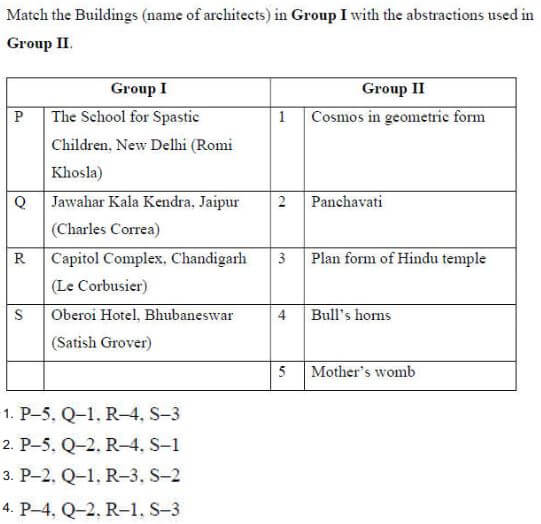Q56.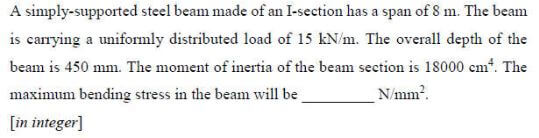Solution: Bending Stress = My/I
Where,
M = Bending Moment
I = Moment of Inertia (I = bd3/12 for a rectangular beam section of area bd)
y = Distance from neutral axis ( y = d/2 of depth d)

Q57. (MSQ Type)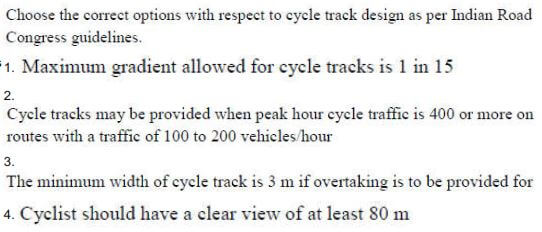Answer: (2) & (3)

Q58.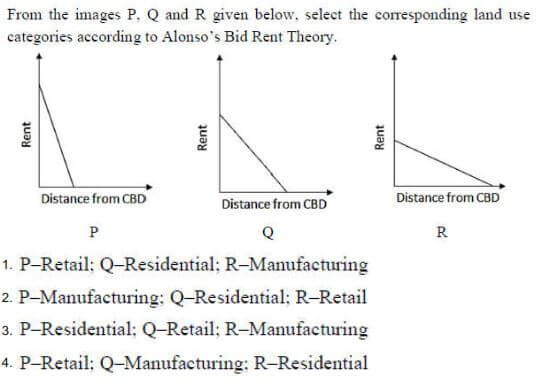Answer: (4)

Q59.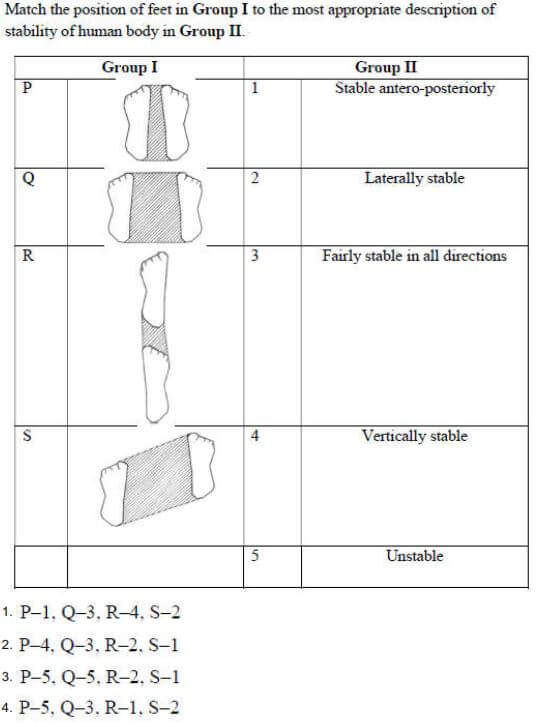Answer: (1)

Q60.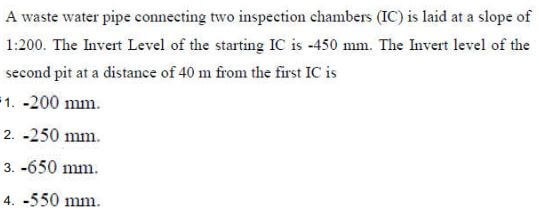Q61.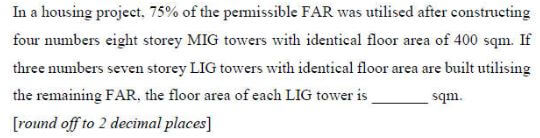Q62.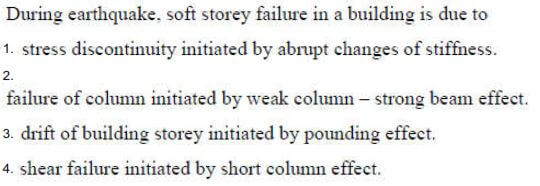Answer: (4)

Q63.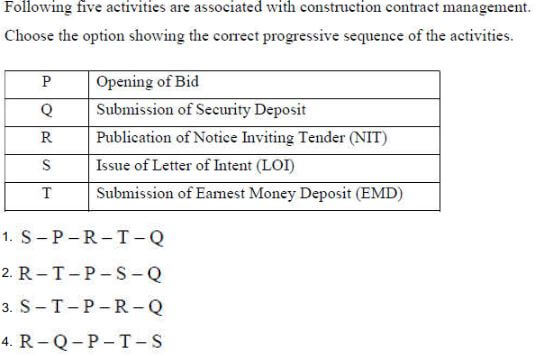Answer: (2)

Q64.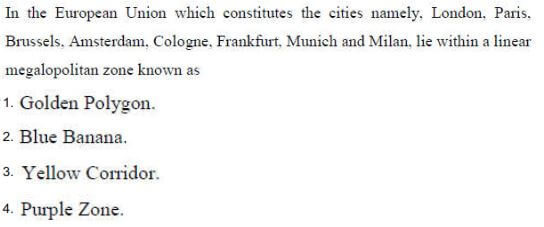Answer: (2)

Q65. (MSQ Type)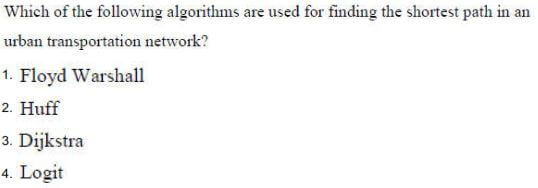This page is created on 6th February 2021.

### 11 Responses

1.Lalit says:

I scored 64.33, what rank should i expect? any idea?

•Vinisha says:

Whats the score now

•Biswajeet says:

70.0

•Akhil says:

Hii

2.HIMANSHU SRIVASTAVA says:

NO. OF TILES USED?

IT SHOULD BE 80 (ANY ANSWER PLEASE)

•Jayati says:

That ans will be 98.
Total no of tiles coming as 104
Flush door contain 6 tiles area.
So (104-6) =98 is the ans

3.HariChaya says:

Is the answer key released all okay? Did anybody find any errors in the answers?

•Subba reddy says:

I think column slenderness ration is 20 not 40

•HariChaya says:

No that is right I think…radius of gyration is d/4….

•Subba reddy says:

Steel stress is 150 not 1.5

4.Prasenjit says:

Mirror image,reverberation,tiles numerical, Jewish berlin museum, lumens numerical ( stadium light),temple architecture, max bending stress,thermal comfort index, Gordon Cullen ,bid rent theory,solar gain factor,landscape,